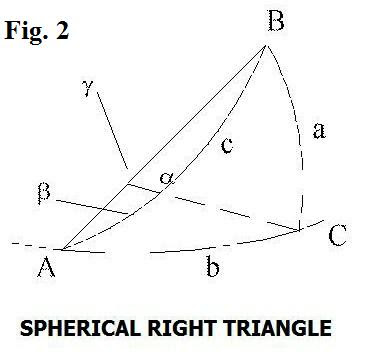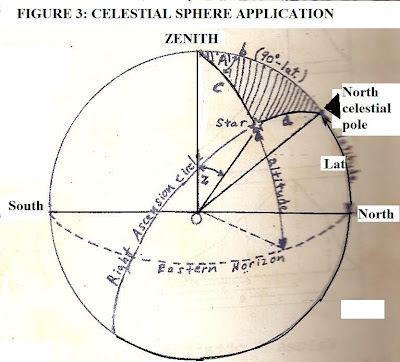## Thursday, March 15, 2018

### Selected Questions -Answers From All Experts Astronomy Forum (Astronomical Coordinates)

Question: I am interested in how astronomical coordinates and angles are computed and the geometry involved. Also can you show an example of how one can calculate a star's declination, say, using known angles?

The sub-discipline to which you refer, computing astronomical coordinates, including in differing coordinate systems, is called "practical astronomy".  The term implies little or no theorization just straight out, bare knuckle observations and mathematical computations.  Practical astronomy entails learning about the mechanics of the sky: how to measure angles and reference coordinates, then how to use these to find astronomical objects in terms of their positions, including altitude for the observer, as well as azimuth.

But before one can do all those things, one has to become au fait with the basic sky coordinate systems and geometry, ultimately working in the basic relations for spherical trigonometry. This is merely an extension of plane trig, but to the sort of angles (many > 90 degrees) one finds in spherical or astronomical applications.

A simple illustration of a spherical geometry is shown in Fig. 1. In the diagram, the angle Θ denotes the longitude measured from some defined meridian on the sphere, while the angle φ denotes a zenith distance, or the measured angle from an object to the zenith.Fig. 2 shows a spherical right triangle from which a host of different angle relationships can be obtained, which can then be used to find astronomical measurements, etc.Fig.3 shows an actual example of a celestial sphere, such as used in many practical astronomy applications, and some of the key angles with reference to a particular object (star) referenced within a given coordinate system. In some applications, the coordinate system may not need to be changed, but in others it must - for example, when going from the coordinate system applied to sky objects (Right Ascension, Declination) to the observer's own coordinates (altitude, azimuth). In this way, coordinate transformations will also enter and we'll get to those in time.For now, let's just consider a simply angle relation in Fig. 1, to find the altitude, a. Then if we have the basic geometrical relationship: a + φ = 90 degrees, clearly then a = (90 - φ ).

Let's now examine Fig. 2 and see what spherical trig relationships we can infer.

Two of the key ones embody the law of sines and law of cosines for spherical triangles, which are the analogs of the law of sines and cosines in plane trig.

We have for the law of sines:

Sin A/ sin a = sin B/ sin b = sin C/ sin c

where A, B, C denote ANGLES and a,b,c denote measured arcs. (Note: we could also have written these by flipping the numerators and denominators).

We have for the law of cosines:

cos a = cos b cos c + sin b sin c cos A

Where a, b, c have the same meanings, and of course, we could write the same relationship out for any included angle.

Now, we use Fig. 3, for a celestial sphere application, in which we use the spherical trig relations to obtain an astronomical measurement.

Using the angles shown in Fig. 3 each of the angles for the law of cosines (given above) can be found. They are as follows:

cos a = cos (90 deg - decl.)

where decl. = declination

cos b = cos (90 deg - Lat)

where 'Lat' denotes the latitude. (Recall from Fig. 1 if φ is polar distance (which can also be zenith distance) then φ = (90 - Lat))

cos c = cos z

where z here is the zenith distance.

sin b = sin (90 deg - Lat)

sin c = sin z

Let's say we want to find the declination of the star if the observer's latitude is 45 degrees N, the azimuth of the star is measured to be 60 degrees, and its zenith distance z = 30 degrees. Then one would solve for cos a:

cos a = = cos (90 deg - decl.)=

cos (90 deg - Lat) cos z + sin (90 deg - Lat) sin z cos (A)

cos (90 deg - decl.)=

cos (90 - 45) cos 30 + sin (90 - 45) sin 30 cos 60

And:

cos (90 deg - decl.)= cos (45) cos 30 + sin (45) sin 30 cos 60

We know, or can use tables or calculator to find:

cos 45 =  Ö2/ 2

cos 30 = Ö3/ 2

sin 45 = Ö2/ 2

sin 30 = ½

cos 60 = ½

Then:

cos (90 deg - decl.)= {(Ö2/ 2 )(Ö3/ 2)} + {Ö2/ 2} (½) (½)

cos (90 deg - decl.)= Ö6/ 4 + Ö2/ 8

= {2 Ö6 +  Ö2/ 8)

cos (90 deg - decl.)= 0.789

arc cos (90 deg - decl.)= 37.9 deg

Then:

decl. = 90 deg - 37.9 deg = 52.1 deg

Or, in more technical terms:

decl. (star) = + 52.1 degrees

As can be seen with this example, once the basic geometry of the sky is grasped, relatively straightforward calculations can be used to obtain various astronomical angular measures as well as coordinates.